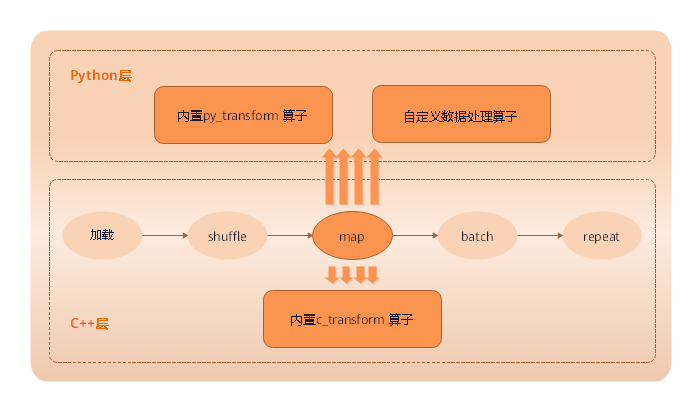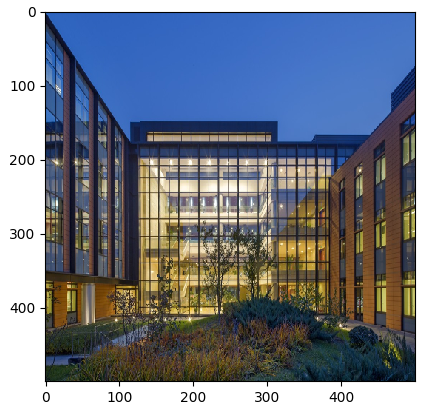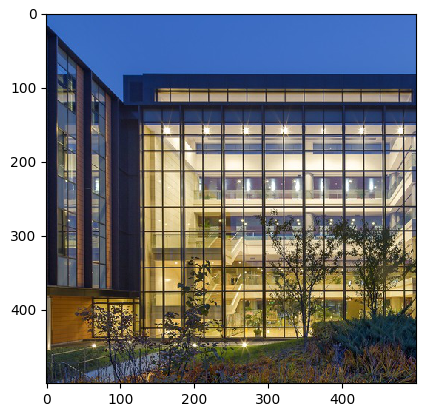# 数据处理与数据增强¶

## MindSpore支持的数据处理操作¶

MindSpore支持多种处理数据操作，包括复制、分批、洗牌、映射等等，详细见下表：

repeat 对数据集进行复制，来加倍数据量。
batch 将数据分批处理，有益于加速训练过程。
shuffle 对数据进行混洗。
map 将提供的函数或算子作用于指定的列数据。
zip 将多个数据集合并成一个数据集。```import mindspore.dataset as ds

ds1 = ds.MnistDataset(MNIST_DATASET_PATH, MNIST_SCHEMA)  # Create MNIST dataset.

ds1 = ds1.shuffle(buffer_size=10000)
ds1 = ds1.batch(32, drop_remainder=True)
ds1 = ds1.repeat(10)
```

1. 导入数据处理操作依赖的模块。

```import mindspore.dataset as ds
```
2. 定义一个生成数据集的函数——`generator_func()`用于生成数据集。

``` def generator_func():
for i in range(5):
yield (np.array([i, i+1, i+2]),)
```
3. 通过`GeneratorDataset`创建`ds1`数据集，用于执行数据处理操作。

```ds1 = ds.GeneratorDataset(generator_func, ["data"])
print("ds1:")
for data in ds1.create_dict_iterator():
print(data["data"])
```

输出：

```ds1:
[0 1 2]
[1 2 3]
[2 3 4]
[3 4 5]
[4 5 6]
```

### repeat¶```def repeat(self, count=None):
```

```ds2 = ds.GeneratorDataset(generator_func, ["data"])
ds2 = ds2.repeat(2)
print("ds2:")
for data in ds2.create_dict_iterator():
print(data["data"])
```

```ds2:
[0 1 2]
[1 2 3]
[2 3 4]
[3 4 5]
[4 5 6]
[0 1 2]
[1 2 3]
[2 3 4]
[3 4 5]
[4 5 6]
```

### batch¶```def batch(self, batch_size, drop_remainder=False, num_parallel_workers=None)
```

• 第1个数据集`ds2`，每2条数据为1组。

• 第2个数据集`ds3`，每3条数据为1组，并将不足1组的数据截掉。

`ds2`示例代码如下：

```ds2 = ds1.batch(batch_size=2)  # Default drop_remainder is False, the last remainder batch isn't dropped.
print("batch size:2    drop remainder:False")
for data in ds2.create_dict_iterator():
print(data["data"])
```

```batch size:2    drop remainder:False
[[0 1 2]
[1 2 3]]
[[2 3 4]
[3 4 5]]
[[4 5 6]]
```

`ds3`示例代码如下：

```ds3 = ds1.batch(batch_size=3, drop_remainder=True)  # When drop_remainder is True, the last remainder batch will be dropped.
print("batch size:3    drop remainder:True")
for data in ds3.create_dict_iterator():
print(data["data"])
```

```batch size:3    drop remainder:True
[[0 1 2]
[1 2 3]
[2 3 4]]
```

### shuffle¶shuffle操作主要用来将数据混洗，设定的buffer_size越大，混洗程度越大，但时间、计算资源消耗会大。 `shuffle()`定义如下：

```def shuffle(self, buffer_size):
```

```print("Before shuffle:")
for data in ds1.create_dict_iterator():
print(data["data"])

ds2 = ds1.shuffle(buffer_size=5)
print("After shuffle:")
for data in ds2.create_dict_iterator():
print(data["data"])
```

```Before shuffle:
[0 1 2]
[1 2 3]
[2 3 4]
[3 4 5]
[4 5 6]
After shuffle:
[3 4 5]
[2 3 4]
[4 5 6]
[1 2 3]
[0 1 2]
```

### map¶

map（映射）即对数据进行处理，譬如将彩色图片的数据集转化为灰色图片的数据集等，应用非常灵活。 MindSpore提供`map()`函数对数据集进行映射操作，用户可以将提供的函数或算子作用于指定的列数据。`map()`函数定义如下：

```def map(self, input_columns=None, operations=None, output_columns=None, columns_order=None,
num_parallel_workers=None):
```

```func = lambda x : x*2  # Define lambda function to multiply each element by 2.
ds2 = ds1.map(input_columns="data", operations=func)
for data in ds2.create_dict_iterator():
print(data["data"])
```

```[0 2 4]
[2 4 6]
[4 6 8]
[6 8 10]
[8 10 12]
```

### zip¶

MindSpore提供`zip()`函数，可将多个数据集合并成1个数据集。

```def zip(self, datasets):
```
1. 采用前面构造数据集`ds1`的方法，构造1个数据集`ds2`

```def generator_func2():
for i in range(5):
yield (np.array([i-3, i-2, i-1]),)

ds2 = ds.GeneratorDataset(generator_func2, ["data2"])
```
2. 通过`zip()`将数据集`ds1``data1`列和数据集`ds2``data2`列合并成数据集`ds3`

```ds3 = ds.zip((ds1, ds2))
for data in ds3.create_dict_iterator():
print(data)
```

输出如下所示：

```{'data1': array([0, 1, 2], dtype=int64), 'data2': array([-3, -2, -1], dtype=int64)}
{'data1': array([1, 2, 3], dtype=int64), 'data2': array([-2, -1,  0], dtype=int64)}
{'data1': array([2, 3, 4], dtype=int64), 'data2': array([-1,  0,  1], dtype=int64)}
{'data1': array([3, 4, 5], dtype=int64), 'data2': array([0, 1, 2], dtype=int64)}
{'data1': array([4, 5, 6], dtype=int64), 'data2': array([1, 2, 3], dtype=int64)}
```

## 数据增强¶

MindSpore提供`c_transforms`模块以及`py_transforms`模块函数供用户进行数据增强操作，用户也可以自定义函数或者算子进行数据增强。MindSpore提供的两个模块的简要说明如下表，详细的介绍请参考API中对应模块的说明。

`c_transforms` 基于C++的OpenCV实现 具有较高的性能。
`py_transforms` 基于Python的PIL实现 该模块提供了多种图像增强功能，并提供了PIL Image和numpy数组之间的传输方法。

### 使用`c_transforms`模块进行数据增强¶

1. 将该模块引入进代码。

```import mindspore.dataset.transforms.vision.c_transforms as transforms
import matplotlib.pyplot as plt
import matplotlib.image as mpimg
```
2. 定义数据增强算子，以`Resize`为例：

```dataset = ds.ImageFolderDatasetV2(DATA_DIR, decode=True)  # Deocde images.
resize_op = transforms.Resize(size=(500,500), interpolation=Inter.LINEAR)
dataset.map(input_columns="image", operations=resize_op)

for data in dataset.create_dict_iterator():
imgplot_resized = plt.imshow(data["image"])
plt.show()
```### 使用`py_transforms`模块进行数据增强¶

1. 将该模块引入到代码。

```import mindspore.dataset.transforms.vision.py_transforms as transforms
import matplotlib.pyplot as plt
import matplotlib.image as mpimg
```
2. 定义数据增强算子，通过`ComposeOp`接口将多个数据增强组合使用, 以`RandomCrop`为例：

```dataset = ds.ImageFolderDatasetV2(DATA_DIR)

transforms_list = [
transforms.Decode(),  # Decode images to PIL format.
transforms.RandomCrop(size=(500,500)),
transforms.ToTensor()  # Convert PIL images to Numpy ndarray.
]
compose = transforms.ComposeOp(transforms_list)
dataset = dataset.map(input_columns="image", operations=compose())
for data in dataset.create_dict_iterator():
print(data["image"])
imgplot_resized = plt.imshow(data["image"].transpose(1, 2, 0))
plt.show()
```